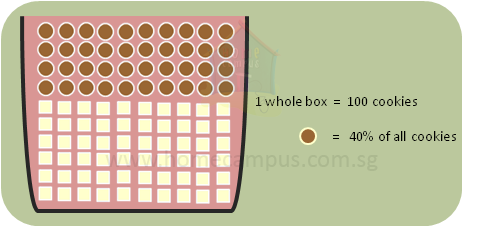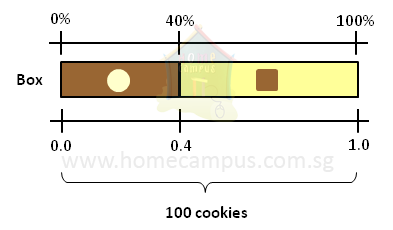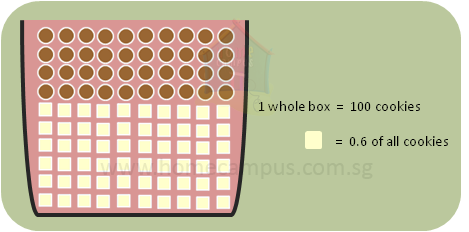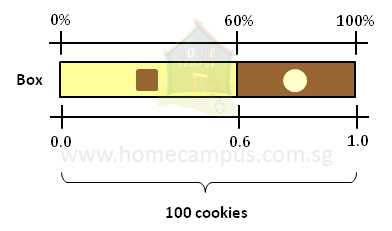## How to Convert Percentage to Decimals and Decimals to Percentage?

Practice Unlimited Questions

#### Converting a percent value to a decimal number and vice versa

Percentages and decimals are related to each other and can be converted from one form to the other.
The video tutorial above explains the steps in detail.

#### 1.  Following is a cookie box containing 100 assorted cookies of 2 different shapes. 40% of the whole box of cookies is round-shaped cookies. Convert this percentage to a decimal.If the whole box of cookies has a value of 100% then 40% of that is round cookies.40%  =
 40 100

=  40  ÷  100
0.4
If the whole box of cookies has a decimal value of 1.0, then 0.4 of it is round cookies.
Using percent value:
=
 40 1001

Using decimal value:

Quick trick:
To convert a percentage to decimal, simply divide the number by 100.

#### 2.  Following is a cookie box containing 100 assorted cookies of 2 different shapes. 0.6 of the whole box of cookies is square-shaped cookies. Convert this decimal number to a percentage.If the whole box of cookies has a value of 1.0 then 0.6 of that is square-shaped cookies.0.6  =  6  ÷  10
=
 6 10

=
 60 100

=  60%
If the whole box of cookies has a percent value of 100%, then 60% of it is square-shaped cookies.
Using decimal value: